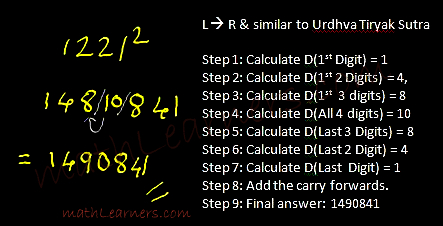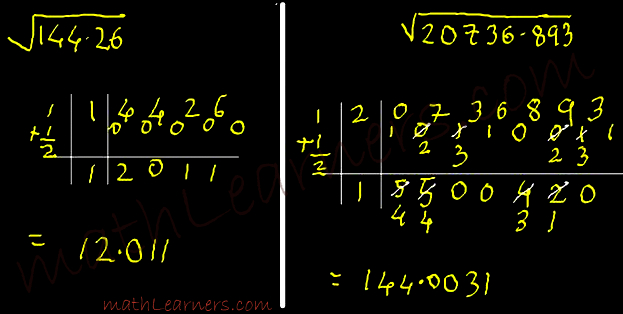### Vedic Maths Coaching in Hisar

Vedic Maths Coaching in Hisar — Accent Coaching Institute Hisar is the Best Coaching Institute in Hisar for VEDIC MATHS COACHING useful for your kids………….MAKE YOUR CHILD FASTER THAN CALCULATORFOR MORE PLEASE CALL: 95410-79129, 96716-39776

–Vedic Mathematics is a collection of Techniques/Sutras to solve mathematical arithmetics in easy and faster way. It consists of 16 Sutras (Formulae) and 13 sub-sutras (Sub Formulae) which can be used for problems involved in arithmetic, algebra, geometry, calculus, conics.

–Vedic Mathematics is a system of mathematics which was discovered by Indian mathematician Jagadguru Shri Bharathi Krishna Tirthaji in the period between A.D. 1911 and 1918 and published his findings in a Vedic Mathematics Book by Tirthaji Maharaj
–Veda is a Sanskrit word which means ‘Knowledge’.

–Using regular mathematical steps, solving problems sometimes are complex and time consuming. But using Vedic
–Mathematic’s General Techniques (applicable to all sets of given data) and Specific Techniques (applicable to specific sets of given data), numerical calculations can be done very fast.

## History Of Vedic Mathematics:

hri Bharathi Krishna Tirthaji Maharaj was born in March 1884 in the Puri village of Orissa state. He was very good in subjects like mathematics, science, humanities and was excellent in Sanskrit language. His interests were also in spiritualism and mediation. In fact when he was practicing meditation in the forest near Sringeri, he rediscovered the Vedic sutras. He claims that these sutras/techniques he learnt from the Vedas especially ‘Rig-Veda’ directly or indirectly and he intuitively rediscovered them when he was practicing meditation for 8 years.

Later he wrote the sutras on the manuscripts but were lost. Finally in year 1957, he wrote introductory volume of 16 sutras which is called as Vedic Mathematics and planned to write other sutras later. But soon he developed cataract in both of his eyes and passed away in year 1960.

## How Vedic Mathematics is Beneficial and What are the Advantages of Vedic Mathematics

Vedic Mathematics can definitely solve mathematical numerical calculations in faster way. Some Vedic Math Scholars mentioned that Using Vedic Maths tricks you can do calculations 10-15 times faster than our usual methods. I agree this to some extent because some methods in Vedic Mathematics are really very fast. But some of this methods are dependent on the specific numbers which are to be calculated. They are called specific methods.

Lets take 1 example to see the Power of Vedic Mathematics.

#### Division Shortcuts in Vedic Mathematics:

1/19 is a Rational Number which forms a recurring decimal number and which recurs the sequence after every 18 digits.

How much time will you take to divide 1/19. Using Ekadhikena Purvena Sutra of Vedic Mathematics, It would take just 7-8 seconds to calculate exact decimal number in just 1 line.Like this, I have mentioned more Divisions in Vedic Mathematics

Gaurav Tekriwal, Founder of www.vedicmathsindia.org, on Quora, mentioned below The Use of Vedic Mathematics.

• More than 1700% times faster than normal Math: this makes it the World’s Fastest.
• Eradicates fear of Math completely. So If your child has Math-Phobia High Speed Vedic Math is a Fun-Filled way to do Math and arises interest in your child.
• Much Improved Academic Performance in School and Instant Results. Just see the first exercise and believe it for yourself. Go over the examples given in the tutorials you would be amazed.
• Sharpens your mind, increases mental agility and intelligence.
• Increases your speed and accuracy. Become a Mental Calculator yourself.
• Improves memory and boosts self confidence.
• Cultivates an Interest in your for numbers.
• Develops your left and right sides of your brain hence using intuition and innovation. It has been noted that Geniuses have been using the right side of the brain to achieve exceptional results.
• Easy to master and apply. You just need the knowledge of tables to learn this.

Vedic Maths Techniques/Sutras have the maths tricks for fast calculation and can be used in exams like CAT, CET, SAT, Banking Exams, etc.

Let’s have a look at some of the techniques used in Vedic Mathematics.

#### Multiplication Techniques/Shortcuts Using Vedic Mathematics:

Using Vedic Maths Tricks you can multiply 962 and 998 in mind in couple of seconds.Vedic Maths Coaching in Hisar is available at Accent Coaching Institute Hisar Haryana

#### Calculating Squares in Vedic Mathematics

How much time will you take to calculate square of 1221.

Using DvandaYoga of Vedic Mathematics, it is just 2 step answer#### Calculating Squares Roots in Vedic Mathematics

How would calculate Square Root of 20736.893?? Using Vedic Mathematics its 4 step method.